Home > CCA2 > Chapter 12 > Lesson 12.1.1 > Problem12-7

12-7.
1. Solve for x in each of the following triangles. What methods can be used for finding unknown parts of triangles? Homework Help ✎

1.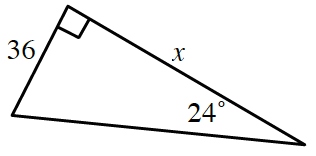2.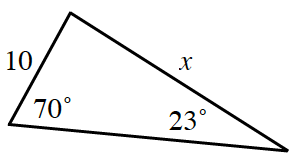3.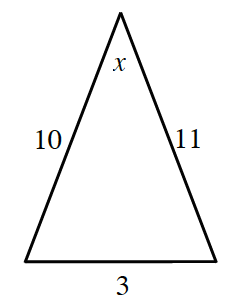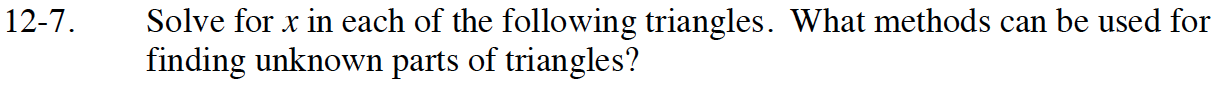What laws and theorems relate the angles of a triangle to the sides?
Or relate the lengths of the sides of a triangle to each other?
Use them to help you find the unknown parts of these triangles.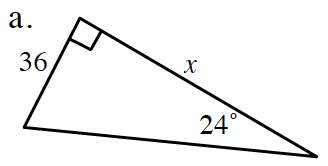Which trigonometric ratio relates the side opposite the angle to the side adjacent to it?

x ≈ 80.86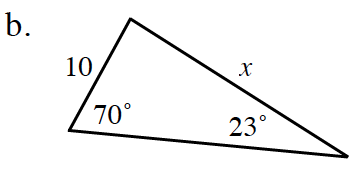Remember that all the angles of a triangle add up to 180°.
Solve for the measure of the unknown angle.
This will help you choose the correct law to solve for the unknown side.

Use the Law of Sines.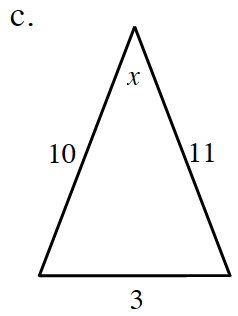Use the Law of Cosines.

x ≈ 15.50°